# Difference between revisions of "Continuous flow"

2010 Mathematics Subject Classification: Primary: 37A10 [MSN][ZBL]

A continuous flow in ergodic theory is a family(whereranges over the real axis) of automorphisms modulo 0 of a measure spacesuch that: a)for any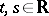and all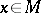, except possibly for a set ofbelonging to an exceptional set of measure 0 (which may depend onand); in other words,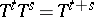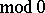; b) for each measurable set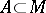the measure of the symmetric differencedepends continuously on. Letbe the set of all automorphisms modulo 0 of the spacewith the usual identification: ifandcoincide almost-everywhere, then they determine the same element of. Ifis endowed with the weak topology (see ), then b) means that the mappingthat takesto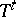is continuous.

If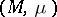is a Lebesgue space, then the concept of a continuous flow is practically the same as that of a measurable flow: The latter is always a continuous flow (see ), and for any continuous flowthere is a measurable flowsuch thatfor all(see ; a related result is proved in , but see also the correction in ). The converse to any of these results depends on the character of the problem in question and the methods used.

In another sense the term "continuous flow" can be used to emphasize that the flow is considered in the context of topological dynamics. In this meaning a continuous flow is a collection of homeomorphismsof a topological spacesuch that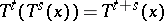for alland; the mappingtaking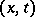tois continuous.

To avoid confusion with 1) it is better to talk in this case of a topological flow and in the case of 1) of a metric continuity.

How to Cite This Entry:
Continuous flow. Encyclopedia of Mathematics. URL: http://encyclopediaofmath.org/index.php?title=Continuous_flow&oldid=15434
This article was adapted from an original article by D.V. Anosov (originator), which appeared in Encyclopedia of Mathematics - ISBN 1402006098. See original article